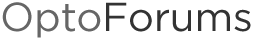# OptoScript to Calculate Dew Point or Relative Humidity

I’m posting this in case it saves time for anyone else. I am using these formulas
to calculate threshold values for a condensation alarm. If the temperature
of a water pipe goes below the calculated dew point temperature of the air,
condensation may occur.

// These algorithms are based on the Magnus-Tetens formula.
// Calculate dew point temperature using measured air temperature
// and measured relative humidity. Air temperature must be between
// 0C and 60C. Relative humidity must be between 1% and 100%
DewPointFactorA = 17.27;
DewPointFactorB = 237.7;
DewPointResultAlpha = (DewPointFactorA * MeasuredAirTempC) / (DewPointFactorB + MeasuredAirTempC) + NaturalLog(MeasuredHumidityPercent / 100);
CalcDewPointValue = (DewPointFactorB * DewPointResultAlpha) / (DewPointFactorA - DewPointResultAlpha);

// Calculate relative humidity using measured air temperature and
// measured dew point temperature. Air temperature must be between
// 0C and 60C. Dew point temperature must be between 0C and 50C
DewPointResultAlpha = MeasuredDewPointTempC * DewPointFactorA - (MeasuredDewPointTempC * DewPointFactorA * MeasuredAirTempC) / (DewPointFactorB + MeasuredAirTempC) - (DewPointFactorB * DewPointFactorA * MeasuredAirTempC) / (DewPointFactorB + MeasuredAirTempC);
CalcHumidityPercent = RaiseEToPower(DewPointResultAlpha / (DewPointFactorB + MeasuredDewPointTempC)) * 100;

Thanks for posting that, Don! This post (click here) includes some other related calculations that might be helpful too.